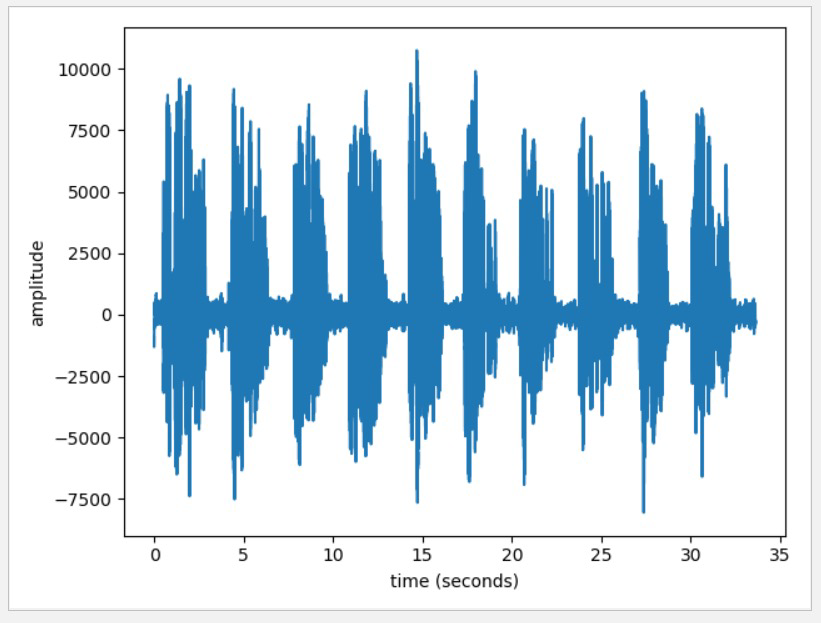# 基于人工智能的语音转波形及波形对比研究

• 时间:
• 浏览:2

1、根据音频转波形技术的实现

import numpy as np

import wave

import pylab as pl

defplot_audio(audio_file='OSR_us_000_0010_8k.wav'):

audio = wave.open(audio_file, 'rb')

params = audio.getparams()

n_channels, _, sample_rate, n_frames = params[:4]

audio.close()

# tranform waveform to array, then plot

time = np.arange(0, n_frames) * (1.0 / sample_rate)

wave_data = np.frombuffer(str_wave_data, dtype=np.short)

if n_channels == 1:

wave_data = np.reshape(wave_data, [-1, 1]).T

pl.subplot(1, 1, 1)

pl.plot(time, wave_data)

pl.xlabel('time (seconds)')

pl.ylabel('amplitude')

pl.show()

elif n_channels == 2:

wave_data = np.reshape(wave_data, [-1, 2]).T

pl.subplot(2, 1, 1)

pl.plot(time, wave_data)

pl.plot(2, 1, 2)

pl.plot(time, wave_data, c='r')

pl.xlabel('time (seconds)')

pl.ylabel('amplitude')

pl.show()

else:

raise ValueError('Only 1 or 2 channels su2、产品技术

3、android

4、 vue

App虽然主要是android开发，但是由于android原生式较为麻烦，为了减少代码量，一些不太重要的地方我们可以用vue来开发。

5、 APi框架

6、python

7、 Springboot